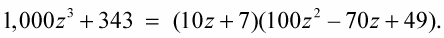##### Linear Algebra For DummiesThe rule for factoring the sum of two perfect cubes is almost the same as the rule for factoring the difference between perfect cubes. You just have to change two little signs to make it work. The sum of two cubes equals the sum of its roots times the squares of its roots minus the product of the roots, which looks like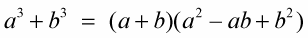Like the results of factoring the difference of two cubes, the results of factoring the sum of two cubes is also made up of:

• A binomial factor (a + b), and

• A trinomial factor,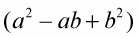Notice that the sign between the two cube roots (a + b) is the same as the sign in the problem to be factored: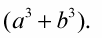The squares in the trinomial expression are still both positive, but you change the sign of the middle term to minus.

Example: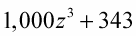Look at this practical example to get a clearer idea of how to factor the sum of two cubes.

• The cube root of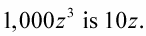• The cube root of 343 is 7.

• The product of 10z and 7 is 70z.

• Thus,